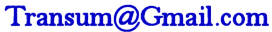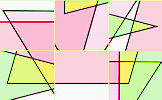# Negative Numbers Level 3

## Use negative numbers in basic arithmetic and algebraic calculations.

##### Level 1Level 2Level 3Level 4Level 5Level 6Level 7DescriptionHelpMore

This is level 3: addition and subtraction You can earn a trophy if you get at least 9 correct and you do this activity online.

 $$-3 + 4$$$$3 + -12$$$$-7 + -12$$$$-8 + -9$$$$-9 + -6$$$$-3 − -2$$$$-1 − 2$$$$-7 − -9$$$$4 − 0$$$$(-7) + (-8) − (-5)$$$$(-4) + (-11) − (-6)$$$$(-9) − (-5) + (-12)$$Check

This is Negative Numbers level 3. You can also try:
Level 1 Level 2 Level 4 Level 5 Level 6 Level 7

Or here are some other negative number exercises to try:
Temperatures Negative Magic Reading Scales Inequalities

## Instructions

Try your best to answer the questions above. Type your answers into the boxes provided leaving no spaces. As you work through the exercise regularly click the "check" button. If you have any wrong answers, do your best to do corrections but if there is anything you don't understand, please ask your teacher for help.

When you have got all of the questions correct you may want to print out this page and paste it into your exercise book. If you keep your work in an ePortfolio you could take a screen shot of your answers and paste that into your Maths file.

## Transum.org

This web site contains over a thousand free mathematical activities for teachers and pupils. Click here to go to the main page which links to all of the resources available.## More Activities:

Mathematicians are not the people who find Maths easy; they are the people who enjoy how mystifying, puzzling and hard it is. Are you a mathematician?

Comment recorded on the 17 June 'Starter of the Day' page by Mr Hall, Light Hall School, Solihull:

"Dear Transum,

I love you website I use it every maths lesson I have with every year group! I don't know were I would turn to with out you!"

Comment recorded on the 19 June 'Starter of the Day' page by Nikki Jordan, Braunton School, Devon:

"Excellent. Thank you very much for a fabulous set of starters. I use the 'weekenders' if the daily ones are not quite what I want. Brilliant and much appreciated."

#### Polygon PiecesArrange the nine pieces of the puzzle on the grid to make the given polygon. Level one is for those learning the names of shapes while other levels are for those who like a challenge!

There are answers to this exercise but they are available in this space to teachers, tutors and parents who have logged in to their Transum subscription on this computer.

A Transum subscription unlocks the answers to the online exercises, quizzes and puzzles. It also provides the teacher with access to quality external links on each of the Transum Topic pages and the facility to add to the collection themselves.

Subscribers can manage class lists, lesson plans and assessment data in the Class Admin application and have access to reports of the Transum Trophies earned by class members.

Subscribe

## Go Maths

Learning and understanding Mathematics, at every level, requires learner engagement. Mathematics is not a spectator sport. Sometimes traditional teaching fails to actively involve students. One way to address the problem is through the use of interactive activities and this web site provides many of those. The Go Maths page is an alphabetical list of free activities designed for students in Secondary/High school.

## Maths Map

Are you looking for something specific? An exercise to supplement the topic you are studying at school at the moment perhaps. Navigate using our Maths Map to find exercises, puzzles and Maths lesson starters grouped by topic.

## Teachers

If you found this activity useful don't forget to record it in your scheme of work or learning management system. The short URL, ready to be copied and pasted, is as follows:

Do you have any comments? It is always useful to receive feedback and helps make this free resource even more useful for those learning Mathematics anywhere in the world. Click here to enter your comments.For All:

© Transum Mathematics :: This activity can be found online at:
www.transum.org/software/SW/Starter_of_the_day/Students/Negative_Numbers.asp?Level=3

## Description of Levels

CloseLevel 1 - Simple subtraction

Example: 5 - 12

Level 2 - Adding to a negative number

Example: -7 + 9

Level 3 - Addition and Subtraction

Example: -10 + -2

Level 4 - Multiplication

Example: -3 x 2

Level 5 - Division

Example: -10 ÷ 5

Level 6 - Substitution

Example: If a=-3 and b=-7 evaluate 7a - 8b - 6

Level 7 - Mixed

Example: A mixed set of word problems

Answers to this exercise are available lower down this page when you are logged in to your Transum account. If you don’t yet have a Transum subscription one can be very quickly set up if you are a teacher, tutor or parent.

### Adding and Subtracting Negative Numbers

The video above is from MathsWatch

### Multiplying and Dividing Negative Numbers

When multiplying or dividing negative numbers remember this:

Same Signs $$\rightarrow$$ Positive Answer

Different Signs $$\rightarrow$$ Negative Answer

Example 1: Same signs (a positive multiplied by a positive) gives a positive answer:

$$12 \times 6 = 72$$

Example 2: Different signs (a positive multiplied by a negative) gives a negative answer:

$$12 \times (-6) = -72$$

Example 3: Different signs (a negative divided by a positive) gives a negative answer:

$$(-12) \div 6 = -2$$

Example 4: Same signs (a negative divided by a negative) gives a positive answer:

$$(-12) \div (-6) = 2$$

Don't wait until you have finished the exercise before you click on the 'Check' button. Click it often as you work through the questions to see if you are answering them correctly. You can double-click the 'Check' button to make it float at the bottom of your screen.

Answers to this exercise are available lower down this page when you are logged in to your Transum account. If you don’t yet have a Transum subscription one can be very quickly set up if you are a teacher, tutor or parent.

Close